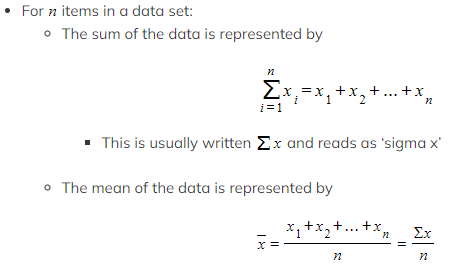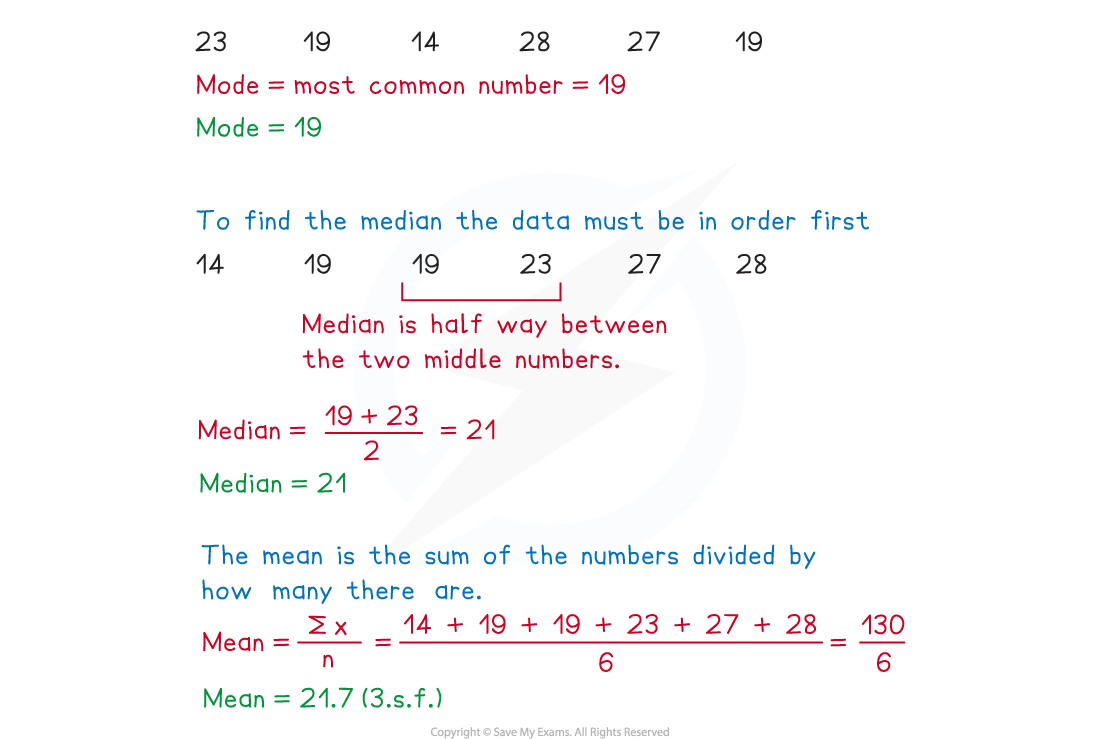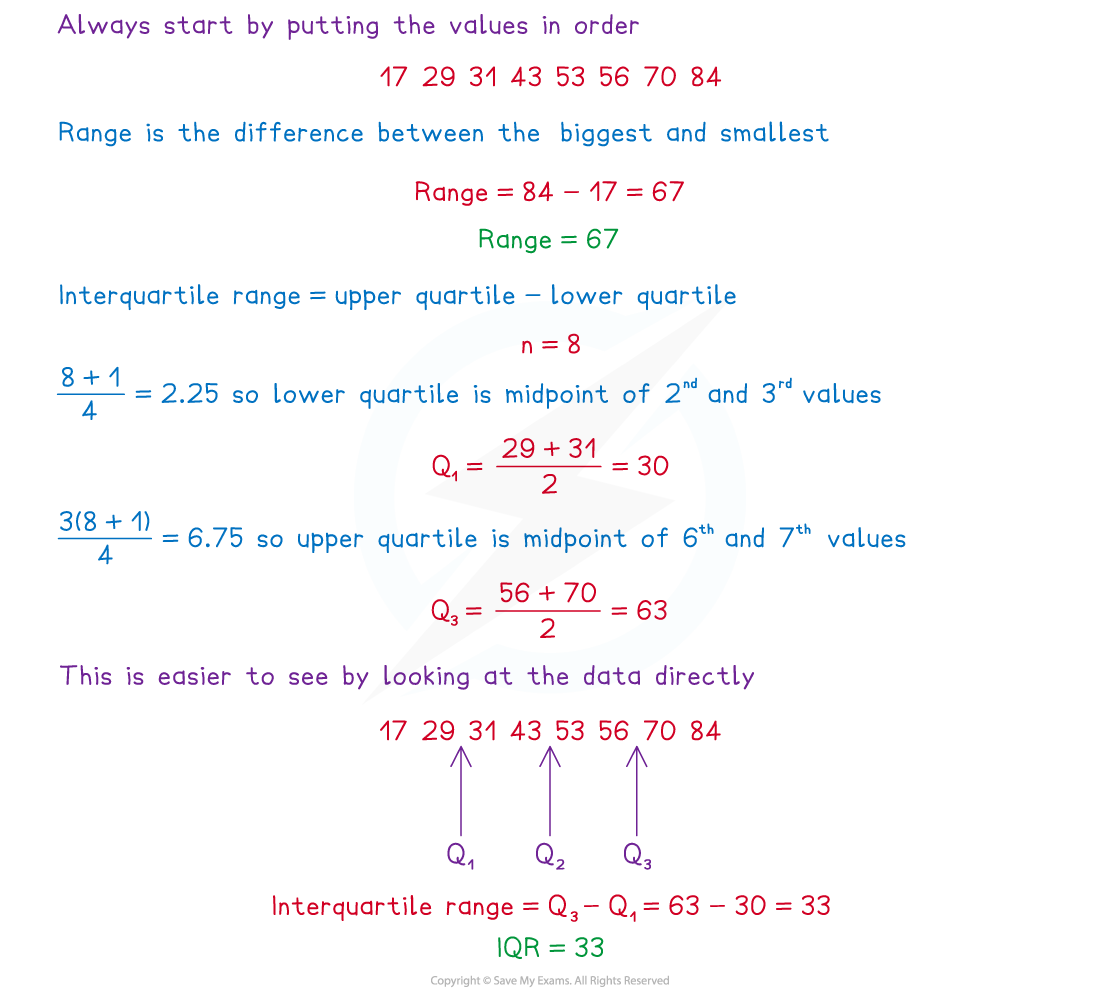# CIE A Level Maths: Probability & Statistics 1复习笔记1.1.1 Basic Statistical Measures

### Types of Data

#### What are the different types of data?

• Qualitative data is data that is usually given in words not numbers to describe something
• For example: the colour of a teacher's car
• Quantitative data is data that is given using numbers which counts or measures something
• For example: the number of pets that a student has
• Discrete data is quantitative data that needs to be counted
• Discrete data can only take specific values from a set of (usually finite) values
• For example: the number of times a coin is flipped until a tails is obtained
• Continuous data is quantitative data that needs to be measured
• Continuous data can take any value within a range of infinite values
• For example: the height of a student
• Age can be discrete or continuous depending on the context or how it is defined
• If you mean how many years old a person is then this is discrete
• If you mean how long a person has been alive then this is continuous

### Mean, Mode, Median

#### What are mean, median and mode?

• Mean, median and mode are measures of location
• A measure of location gives information about where data is in the number system
• Mean, median and mode are measures of central tendency
• They describe where the centre of the data is
• They are all types of averages
• In Statistics it is important to be specific about which average you are referring to

#### How are mean, median and mode calculated?

• You should already be familiar with finding the mean, median and mode from raw, ungrouped data
• The mode is the value that occurs most often in a data set
• In a frequency table the group or class that occurs most often will be referred to as the modal class
• A data set with more than one mode is bimodal
• The median is the middle value when the data is in order of size
• If there are two values in the middle of the data set, the median is the midpoint of the two values
• If finding median from a frequency table find the cumulative frequency first and find the group or class where the middle value will lie
• The mean is the sum of all the values divided by the number of values in the data set

#### What are summary statistics and their notation?

• Summary statistics are information that summarises a set of data values
•This reads as ‘x bar’
• You will come across more summary statistics later in the course

#### How do we choose the best measure of central tendency?

• It is often better to use one of the averages over the others, depending on the data set
• It’s a good idea to be aware of the advantages and disadvantages of the use of each average
• The mean uses all of the data values, this is good for a large data set where all of the values are close together, but also means that the mean can be affected by extreme values
• The median is not affected by very high or low values so is a good average to use in data sets with extreme values
• The mode is very useful in a lot of practical situations, however often there may be more than one mode, no mode or even a mode that is nowhere near the middle of the data set

#### Worked Example

For the data set given below, find the mode, median and mean.

23             19              14             28              27            19### Quartiles & Range

What are quartiles?

• Quartiles and percentiles are measures of location
• Quartilesdivide a populationor data setinto four equal sections

#### What are the range and interquartile range?

• The range and interquartile range are both measures of spread
• A measure of spread gives information about how spread out the data set is
• The range is the difference between the largest and smallest values in the data set
• All data points in the set will be included in the range, including extreme values
• The interquartile range is the difference between the upper quartile and the lower quartile
• Only the middle 50% of the data is included in the interquartile range
• It is not affected by extreme values
• The units for range and interquartile range are the same as the units for the original data

#### Worked Example

Find the range and interquartile range for the data set given below

43             29               70               31               84                56               17               53.#### Exam Tip

• Be aware of the difference between averages and ranges, especially when answering contextual questions asking you to describe or compare data. Remember, averages give an indication of where the data are whilst range gives an indication of how varied the data are.